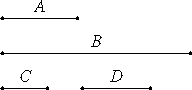# Proposition 3

If a cubic number multiplied by itself makes some number, then the product is a cube.

Let the cubic number A multiplied by itself make B.

I say that B is cubic.

Take C, the side of A. Multiply C by itself make D. It is then manifest that C multiplied by D makes A.VII.Def.20

Now, since C multiplied by itself makes D, therefore C measures D according to the units in itself. But further the unit also measures C according to the units in it, therefore the unit is to C as C is to D.

Again, since C multiplied by D makes A, therefore D measures A according to the units in C. But the unit also measures C according to the units in it, therefore the unit is to C as D is to A. But the unit is to C as C is to D, therefore the unit is to C as C is to D, and as D is to A.

Therefore between the unit and the number A two mean proportional numbers C and D have fallen in continued proportion.

VII.Def.20

Again, since A multiplied by itself makes B, therefore A measures B according to the units in itself. But the unit also measures A according to the units in it, therefore the unit is to A as A is to B.

VIII.8

But between the unit and A two mean proportional numbers have fallen, therefore two mean proportional numbers also fall between A and B.

VIII.23

But, if two mean proportional numbers fall between two numbers, and the first is a cube, then the second is also a cube. And A is a cube, therefore B is also a cube.

Therefore, if a cubic number multiplied by itself makes some number, then the product is a cube.

Q.E.D.

## Guide

Modern algebra certainly makes short work of this proposition: (c3)2 = (c2)3.

#### Use of this proposition

This proposition is used in the next two propositions and IX.9.